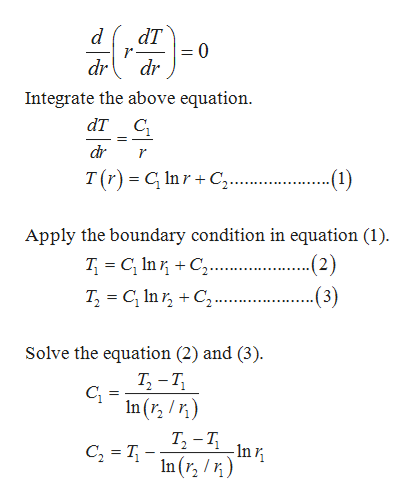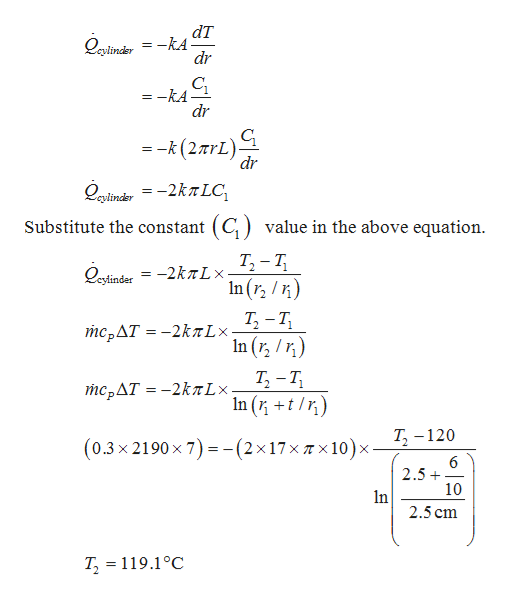Question
307 views

A pipe in a manufacturing plant is transporting superheated vapor at a mass flow rate of 0.3 kg/s. The pipe is 10 m long and has an inner diameter of 5 cm and a wall thickness of 6 mm. The pipe has a thermal conductivity of 17 W/m·K, and the inner pipe surface is at a uniform temperature of 120°C. The temperature drop between the inlet and exit of the pipe is 7°C, and the constant pressure specific heat of vapor is 2190 J/kg·°C. If the air temperature in the manufacturing plant is 25°C, determine the heat transfer coefficient as a result of convection between the outer pipe surface and the surrounding air.

check_circle

Step 1

Write the differential equation for the steady state one dimension heat conduction.help_outlineImage TranscriptionclosedT = 0 dr dr Integrate the above equation. dT C _ dr r -(1) T(r) G InrC.. = Apply the boundary condition in equation (1) -(2) (3) T C In C. T, C In r, C. Solve the equation (2) and (3) T-T In (r, ) T2-11In C2 In(r) fullscreen
Step 2

Write the formula for the  heat ...help_outlineImage TranscriptionclosedT Qxinder = -kA dr cylin CL -kA dr =-k (27rL) dr =-2kTLC cylinder (C value in the above equation Substitute the constant sytinder = -2KT- 12-T In ( ) mcpAT = -2kxLx 2- In (r/) Т, - т, In (t) тсрДT %3 - 2kлLx + T2 -120 (0.3x 2190x 7) -(2x17x Tx 10) x- 6 2.5+ 10 In 2.5 cm T, = 119.1°C fullscreen

### Want to see the full answer?

See Solution

#### Want to see this answer and more?

Solutions are written by subject experts who are available 24/7. Questions are typically answered within 1 hour.*

See Solution
*Response times may vary by subject and question.
Tagged in

### Other